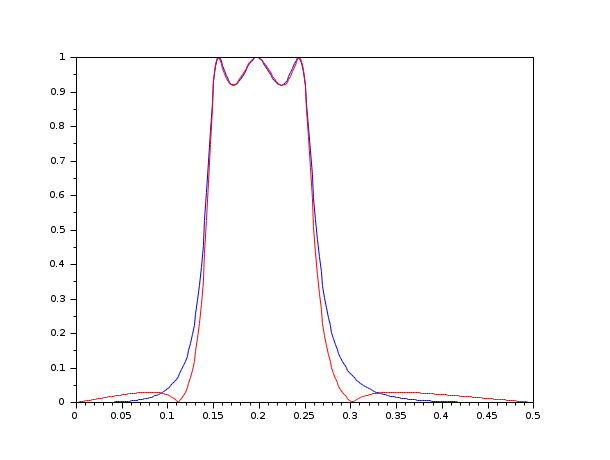Scilab Home page | Wiki | Bug tracker | Forge | Mailing list archives | ATOMS | File exchange
Change language to: Français - Português - 日本語 - Русский

See the recommended documentation of this function

Scilab Help >> Signal Processing > filters > frmag

# frmag

magnitude of FIR and IIR filters

### Calling Sequence

```[xm,fr]=frmag(sys,npts)
[xm,fr]=frmag(num,den,npts)```

### Arguments

sys

a single input, single output discrete transfer function, or a polynomial or the vector of polynomial coefficients, the filter.

num

a polynomial or the vector of polynomial coefficients, the numerator of the filter

den

a polynomial or the vector of polynomial coefficients, the denominator of the filter (the default value is 1).

npts

integer, the number of points in frequency response.

xm

vector of magnitude of frequency response at the points `fr`.

fr

points in the normalized frequency domain where magnitude is evaluated.

### Description

calculates the magnitude of the frequency responses of FIR and IIR filters. The filter description can be one or two vectors of coefficients, one or two polynomials, or a single output discrete transfer function.

the frequency discretization is given by `fr=linspace(0,1/2,npts)`.

### Examples

```hz=iir(3,'bp','cheb1',[.15 .25],[.08 .03]);
[hzm,fr]=frmag(hz,256);
plot(fr,hzm)
hz=iir(3,'bp','ellip',[.15 .25],[.08 .03]);
[hzm,fr]=frmag(hz,256);
plot(fr,hzm,'r')```• iir — iir digital filter
• eqfir — minimax approximation of FIR filter
• repfreq — frequency response
• calfrq — frequency response discretization
• phasemag — phase and magnitude computation